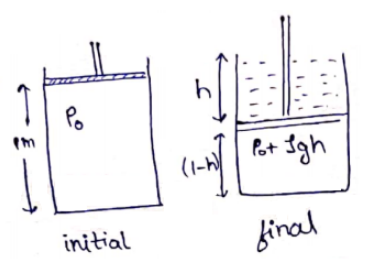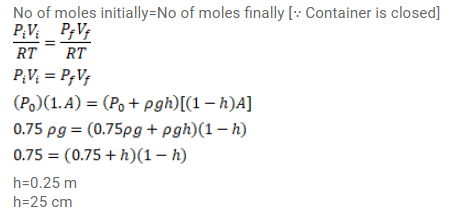# Use R=8.3 J/mol-K wherever required. A vertical cylinder of height 100 cm containsQuestion:

Use $R=8.3 \mathrm{~J} / \mathrm{mol}-\mathrm{K}$ wherever required.

A vertical cylinder of height $100 \mathrm{~cm}$ contains air at a constant temperature. The top is closed by a frictionless light piston. The atmospheric pressure is equal to $75 \mathrm{~cm}$ of mercury. Mercury is slowly poured over the piston. Find the maximum height of the mercury column that can be put on the piston.

Solution: